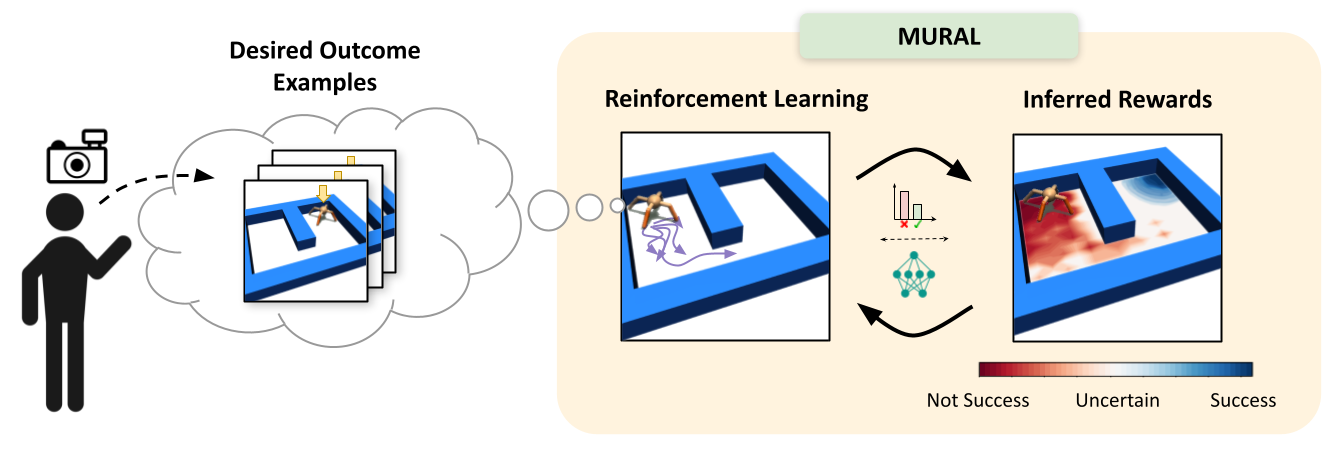Diagram of MURAL, our method for learning uncertainty-aware rewards for RL. After the user provides a few examples of desired outcomes, MURAL automatically infers a reward function that takes into account these examples and the agent’s uncertainty for each state.

Although reinforcement learning has shown success in domains such as robotics, chip placement and playing video games, it is usually intractable in its most general form. In particular, deciding when and how to visit new states in the hopes of learning more about the environment can be challenging, especially when the reward signal is uninformative. These questions of reward specification and exploration are closely connected — the more directed and “well shaped” a reward function is, the easier the problem of exploration becomes. The answer to the question of how to explore most effectively is likely to be closely informed by the particular choice of how we specify rewards.

For unstructured problem settings such as robotic manipulation and navigation — areas where RL holds substantial promise for enabling better real-world intelligent agents — reward specification is often the key factor preventing us from tackling more difficult tasks. The challenge of effective reward specification is two-fold: we require reward functions that can be specified in the real world without significantly instrumenting the environment, but also effectively guide the agent to solve difficult exploration problems. In our recent work, we address this challenge by designing a reward specification technique that naturally incentivizes exploration and enables agents to explore environments in a directed way.

# Outcome Driven RL and Classifier Based Rewards

While RL in its most general form can be quite difficult to tackle, we can consider a more controlled set of subproblems which are more tractable while still encompassing a significant set of interesting problems. In particular, we consider a subclass of problems which has been referred to as outcome driven RL. In outcome driven RL problems, the agent is not simply tasked with exploring the environment until it chances upon reward, but instead is provided with examples of successful outcomes in the environment. These successful outcomes can then be used to infer a suitable reward function that can be optimized to solve the desired problems in new scenarios.

More concretely, in outcome driven RL problems, a human supervisor first provides a set of successful outcome examples ${s_g^i}_{i=1}^N$, representing states in which the desired task has been accomplished. Given these outcome examples, a suitable reward function $r(s, a)$ can be inferred that encourages an agent to achieve the desired outcome examples. In many ways, this problem is analogous to that of inverse reinforcement learning, but only requires examples of successful states rather than full expert demonstrations.

When thinking about how to actually infer the desired reward function $r(s, a)$ from successful outcome examples ${s_g^i}_{i=1}^N$, the simplest technique that comes to mind is to simply treat the reward inference problem as a classification problem - “Is the current state a successful outcome or not?” Prior work has implemented this intuition, inferring rewards by training a simple binary classifier to distinguish whether a particular state $s$ is a successful outcome or not, using the set of provided goal states as positives, and all on-policy samples as negatives. The algorithm then assigns rewards to a particular state using the success probabilities from the classifier. This has been shown to have a close connection to the framework of inverse reinforcement learning.

Classifier-based methods provide a much more intuitive way to specify desired outcomes, removing the need for hand-designed reward functions or demonstrations: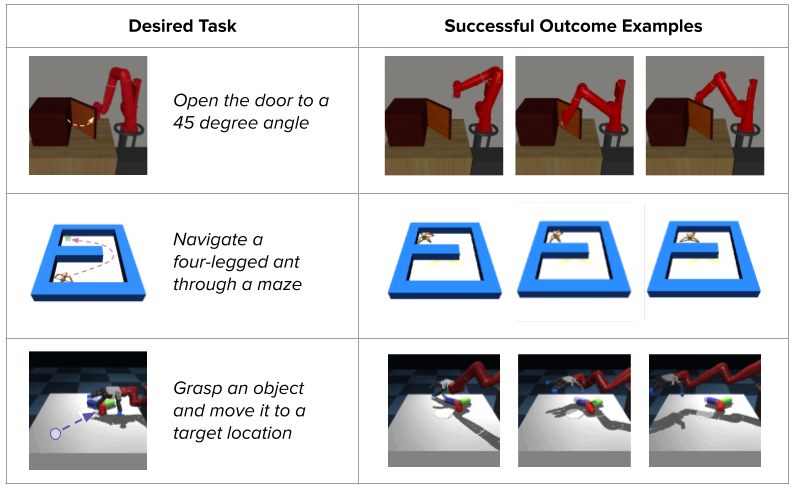These classifier-based methods have achieved promising results on robotics tasks such as fabric placement, mug pushing, bead and screw manipulation, and more. However, these successes tend to be limited to simple shorter-horizon tasks, where relatively little exploration is required to find the goal.

# What’s Missing?

Standard success classifiers in RL suffer from the key issue of overconfidence, which prevents them from providing useful shaping for hard exploration tasks. To understand why, let’s consider a toy 2D maze environment where the agent must navigate in a zigzag path from the top left to the bottom right corner. During training, classifier-based methods would label all on-policy states as negatives and user-provided outcome examples as positives. A typical neural network classifier would easily assign success probabilities of 0 to all visited states, resulting in uninformative rewards in the intermediate stages when the goal has not been reached.

Since such rewards would not be useful for guiding the agent in any particular direction, prior works tend to regularize their classifiers using methods like weight decay or mixup, which allow for more smoothly increasing rewards as we approach the successful outcome states. However, while this works on many shorter-horizon tasks, such methods can actually produce very misleading rewards. For example, on the 2D maze, a regularized classifier would assign relatively high rewards to states on the opposite side of the wall from the true goal, since they are close to the goal in x-y space. This causes the agent to get stuck in a local optima, never bothering to explore beyond the final wall!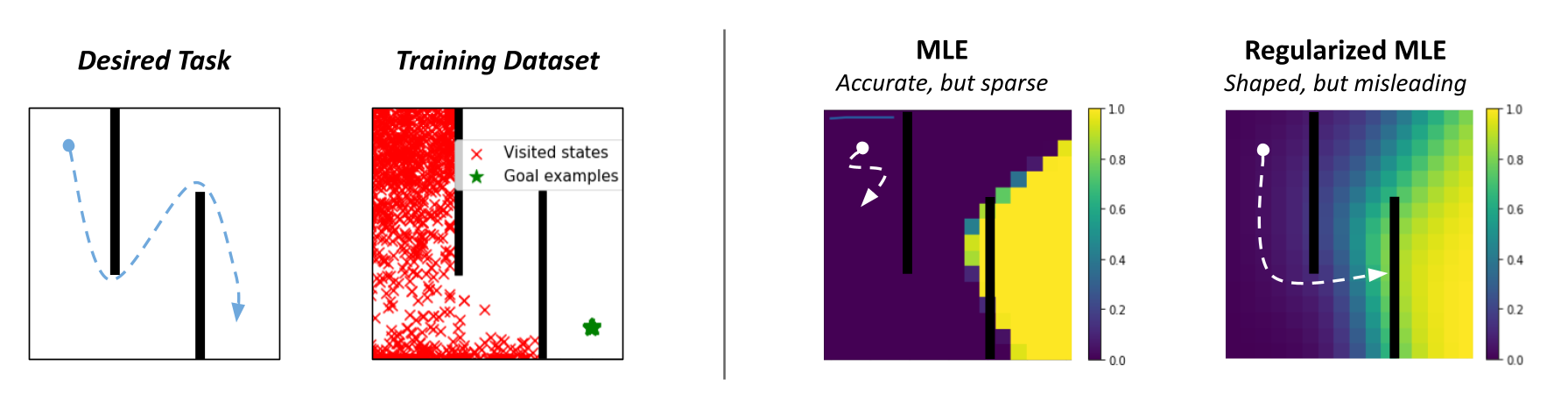In fact, this is exactly what happens in practice: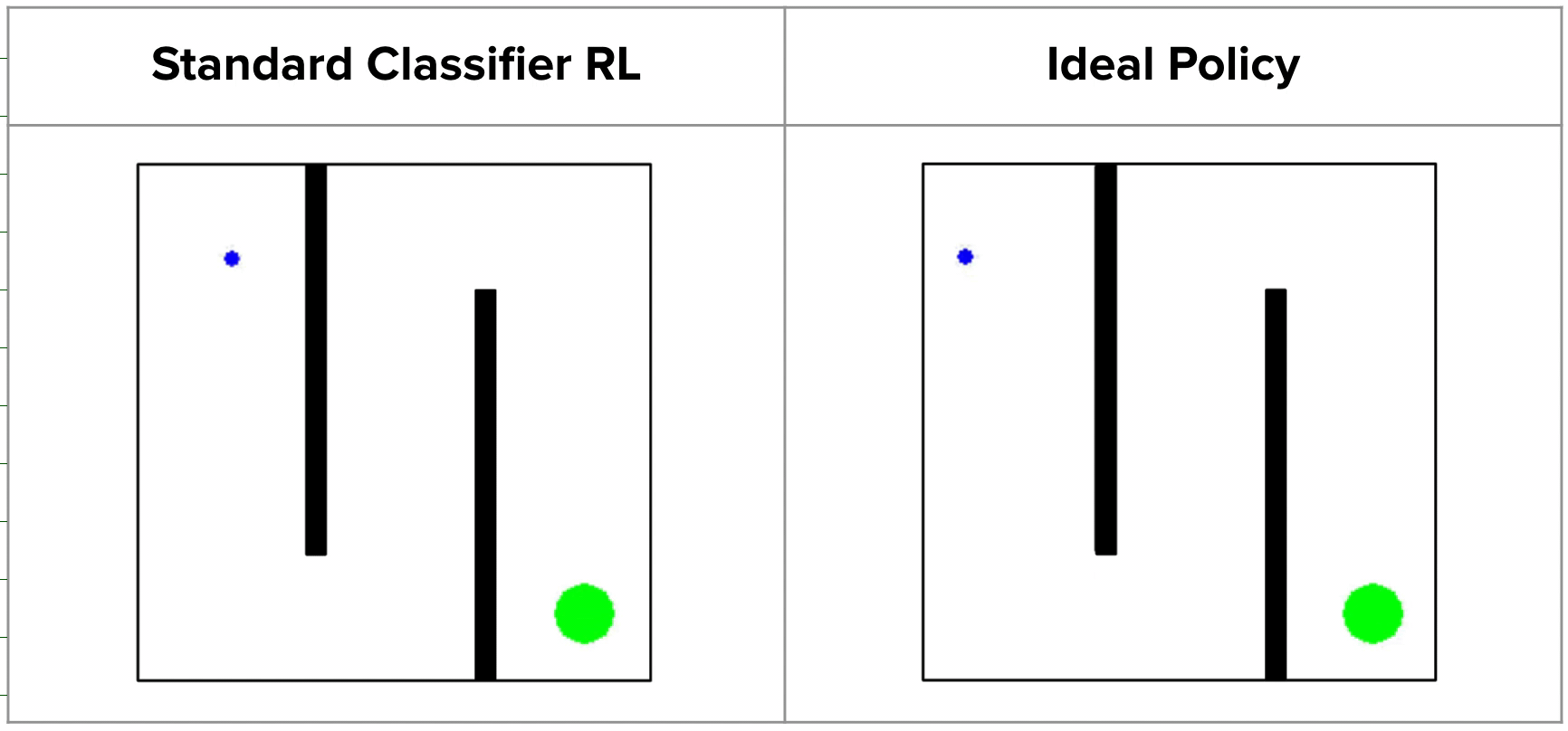# Uncertainty-Aware Rewards through CNML

As discussed above, the key issue with unregularized success classifiers for RL is overconfidence — by immediately assigning rewards of 0 to all visited states, we close off many paths that might eventually lead to the goal. Ideally, we would like our classifier to have an appropriate notion of uncertainty when outputting success probabilities, so that we can avoid excessively low rewards without suffering from the misleading local optima that result from regularization.

Conditional Normalized Maximum Likelihood (CNML)
One method particularly well-suited for this task is Conditional Normalized Maximum Likelihood (CNML). The concept of normalized maximum likelihood (NML) has typically been used in the Bayesian inference literature for model selection, to implement the minimum description length principle. In more recent work, NML has been adapted to the conditional setting to produce models that are much better calibrated and maintain a notion of uncertainty, while achieving optimal worst case classification regret. Given the challenges of overconfidence described above, this is an ideal choice for the problem of reward inference.

Rather than simply training models via maximum likelihood, CNML performs a more complex inference procedure to produce likelihoods for any point that is being queried for its label. Intuitively, CNML constructs a set of different maximum likelihood problems by labeling a particular query point $x$ with every possible label value that it might take, then outputs a final prediction based on how easily it was able to adapt to each of those proposed labels given the entire dataset observed thus far. Given a particular query point $x$, and a prior dataset $\mathcal{D} = \left[x_0, y_0, … x_N, y_N\right]$, CNML solves k different maximum likelihood problems and normalizes them to produce the desired label likelihood $p(y \mid x)$, where $k$ represents the number of possible values that the label may take. Formally, given a model $f(x)$, loss function $\mathcal{L}$, training dataset $\mathcal{D}$ with classes $\mathcal{C}_1, …, \mathcal{C}_k$, and a new query point $x_q$, CNML solves the following $k$ maximum likelihood problems:

$\theta_i = \text{arg}\max_{\theta} \mathbb{E}_{\mathcal{D} \cup (x_q, C_i)}\left[ \mathcal{L}(f_{\theta}(x), y)\right]$

It then generates predictions for each of the $k$ classes using their corresponding models, and normalizes the results for its final output:

$p_\text{CNML}(C_i|x) = \frac{f_{\theta_i}(x)}{\sum \limits_{j=1}^k f_{\theta_j}(x)}$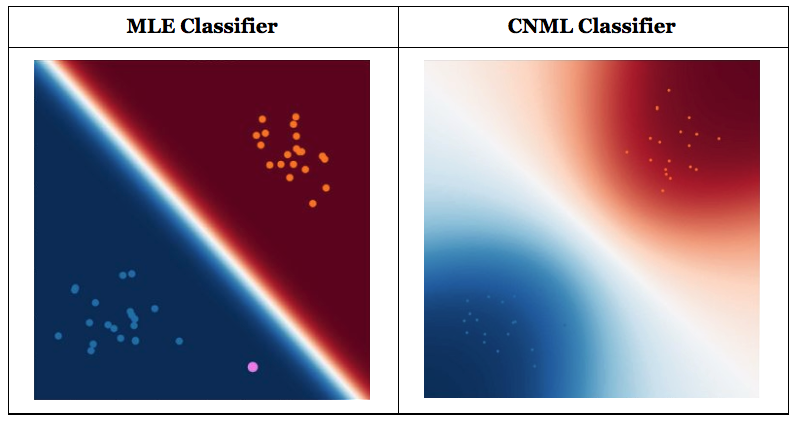Comparison of outputs from a standard classifier and a CNML classifier. CNML outputs more conservative predictions on points that are far from the training distribution, indicating uncertainty about those points’ true outputs. (Credit: Aurick Zhou, BAIR Blog)

Intuitively, if the query point is farther from the original training distribution represented by D, CNML will be able to more easily adapt to any arbitrary label in $\mathcal{C}_1, …, \mathcal{C}_k$, making the resulting predictions closer to uniform. In this way, CNML is able to produce better calibrated predictions, and maintain a clear notion of uncertainty based on which data point is being queried.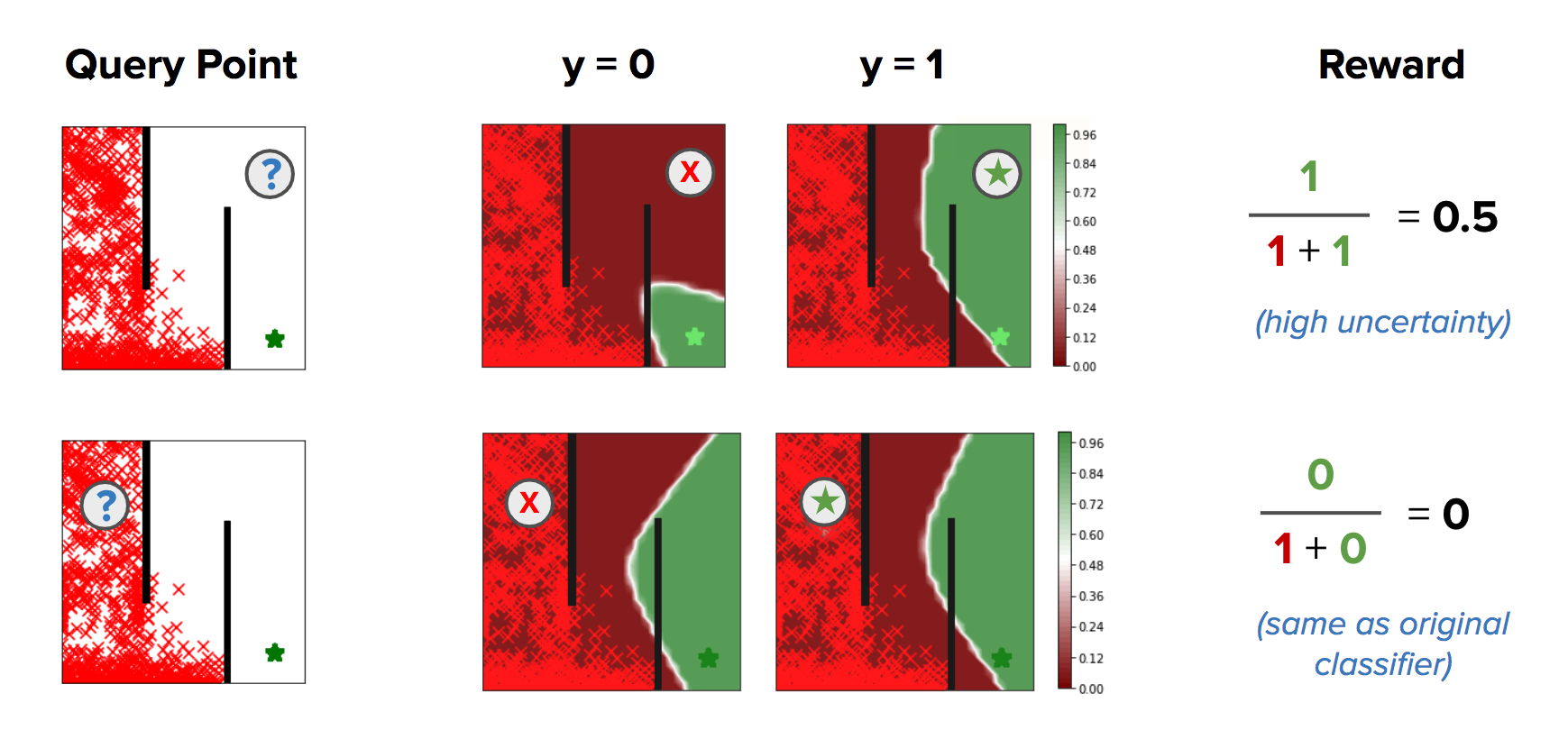Leveraging CNML-based classifiers for Reward Inference
Given the above background on CNML as a means to produce better calibrated classifiers, it becomes clear that this provides us a straightforward way to address the overconfidence problem with classifier based rewards in outcome driven RL. By replacing a standard maximum likelihood classifier with one trained using CNML, we are able to capture a notion of uncertainty and obtain directed exploration for outcome driven RL. In fact, in the discrete case, CNML corresponds to imposing a uniform prior on the output space — in an RL setting, this is equivalent to using a count-based exploration bonus as the reward function. This turns out to give us a very appropriate notion of uncertainty in the rewards, and solves many of the exploration challenges present in classifier based RL.

However, we don’t usually operate in the discrete case. In most cases, we use expressive function approximators and the resulting representations of different states in the world share similarities. When a CNML based classifier is learned in this scenario, with expressive function approximation, we see that it can provide more than just task agnostic exploration. In fact, it can provide a directed notion of reward shaping, which guides an agent towards the goal rather than simply encouraging it to expand the visited region naively. As visualized below, CNML encourages exploration by giving optimistic success probabilities in less-visited regions, while also providing better shaping towards the goal.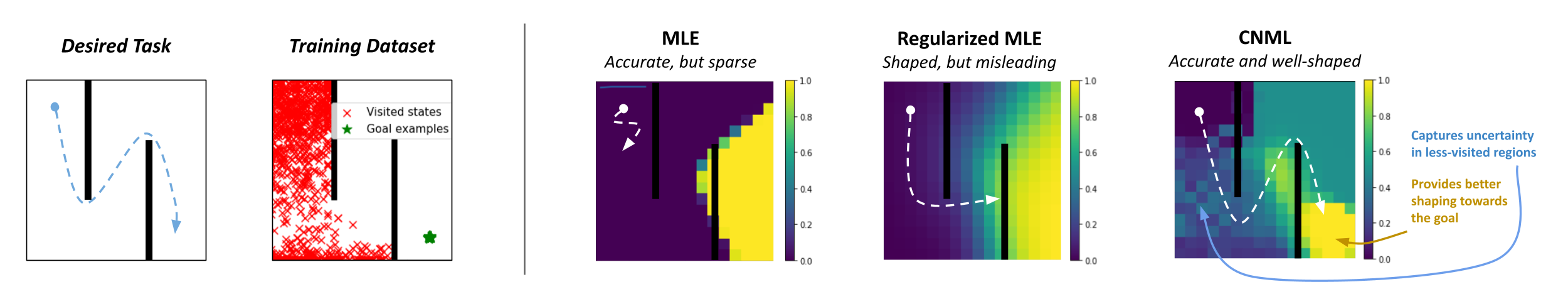As we will show in our experimental results, this intuition scales to higher dimensional problems and more complex state and action spaces, enabling CNML based rewards to solve significantly more challenging tasks than is possible with typical classifier based rewards.

However, on closer inspection of the CNML procedure, a major challenge becomes apparent. Each time a query is made to the CNML classifier, $k$ different maximum likelihood problems need to be solved to convergence, then normalized to produce the desired likelihood. As the size of the dataset increases, as it naturally does in reinforcement learning, this becomes a prohibitively slow process. In fact, as seen in Table 1, RL with standard CNML based rewards takes around 4 hours to train a single epoch (1000 timesteps). Following this procedure blindly would take over a month to train a single RL agent, necessitating a more time efficient solution. This is where we find meta-learning to be a crucial tool.

# Meta-Learning CNML Classifiers

Meta-learning is a tool that has seen a lot of use cases in few-shot learning for image classification, learning quicker optimizers and even learning more efficient RL algorithms. In essence, the idea behind meta-learning is to leverage a set of “meta-training” tasks to learn a model (and often an adaptation procedure) that can very quickly adapt to a new task drawn from the same distribution of problems.

Meta-learning techniques are particularly well suited to our class of computational problems since it involves quickly solving multiple different maximum likelihood problems to evaluate the CNML likelihood. Each the maximum likelihood problems share significant similarities with each other, enabling a meta-learning algorithm to very quickly adapt to produce solutions for each individual problem. In doing so, meta-learning provides us an effective tool for producing estimates of normalized maximum likelihood significantly more quickly than possible before.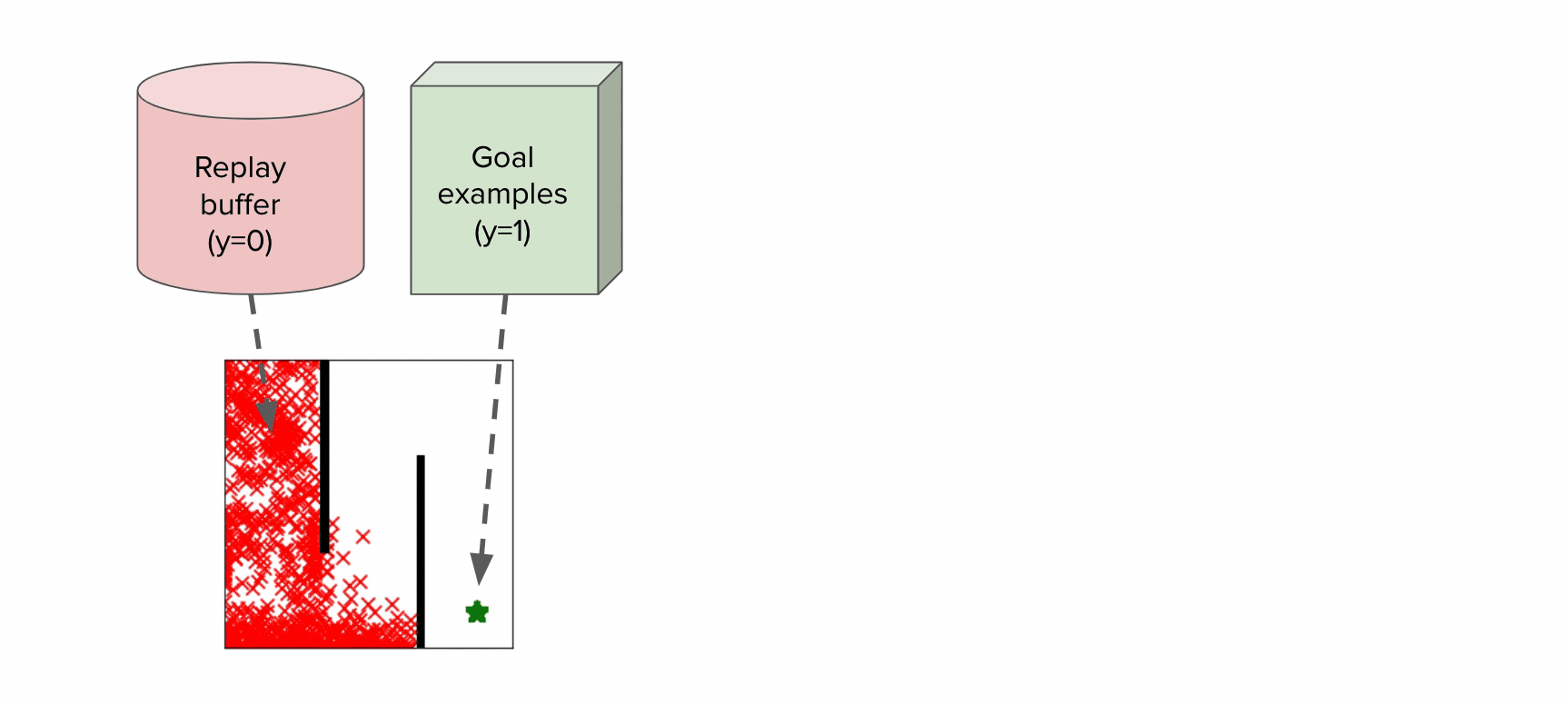The intuition behind how to apply meta-learning to the CNML (meta-NML) can be understood by the graphic above. For a data-set of $N$ points, meta-NML would first construct $2N$ tasks, corresponding to the positive and negative maximum likelihood problems for each datapoint in the dataset. Given these constructed tasks as a (meta) training set, a meta-learning algorithm can be applied to learn a model that can very quickly be adapted to produce solutions to any of these $2N$ maximum likelihood problems. Equipped with this scheme to very quickly solve maximum likelihood problems, producing CNML predictions around $400$x faster than possible before. Prior work studied this problem from a Bayesian approach, but we found that it often scales poorly for the problems we considered.

Equipped with a tool for efficiently producing predictions from the CNML distribution, we can now return to the goal of solving outcome-driven RL with uncertainty aware classifiers, resulting in an algorithm we call MURAL.

# MURAL: Meta-Learning Uncertainty-Aware Rewards for Automated Reinforcement Learning

To more effectively solve outcome driven RL problems, we incorporate meta-NML into the standard classifier based procedure as follows: After each epoch of RL, we sample a batch of $n$ points from the replay buffer and use them to construct $2n$ meta-tasks. We then run $1$ iteration of meta-training on our model. We assign rewards using NML, where the NML outputs are approximated using only one gradient step for each input point.

The resulting algorithm, which we call MURAL, replaces the classifier portion of standard classifier-based RL algorithms with a meta-NML model instead. Although meta-NML can only evaluate input points one at a time instead of in batches, it is substantially faster than naive CNML, and MURAL is still comparable in runtime to standard classifier-based RL, as shown in Table 1 below.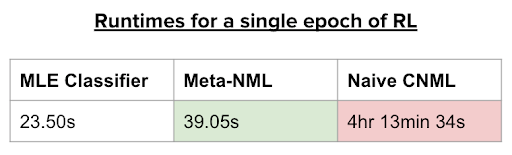Table 1. Runtimes for a single epoch of RL on the 2D maze task.

We evaluate MURAL on a variety of navigation and robotic manipulation tasks, which present several challenges including local optima and difficult exploration. MURAL solves all of these tasks successfully, outperforming prior classifier-based methods as well as standard RL with exploration bonuses.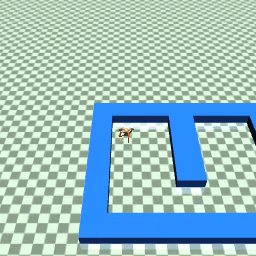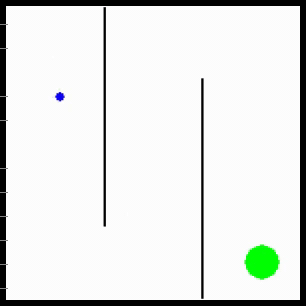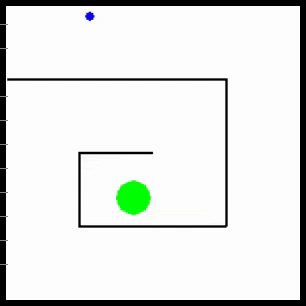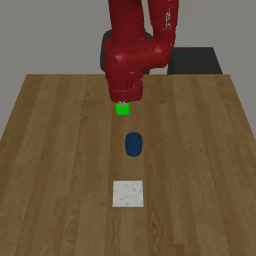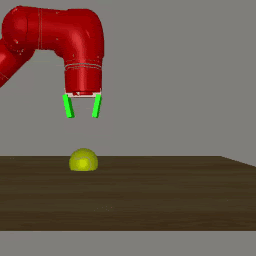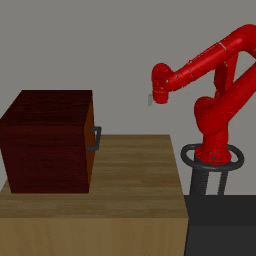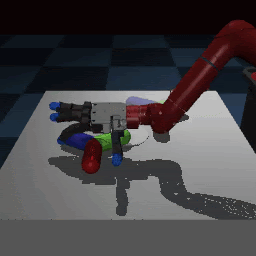Visualization of behaviors learned by MURAL. MURAL is able to perform a variety of behaviors in navigation and manipulation tasks, inferring rewards from outcome examples.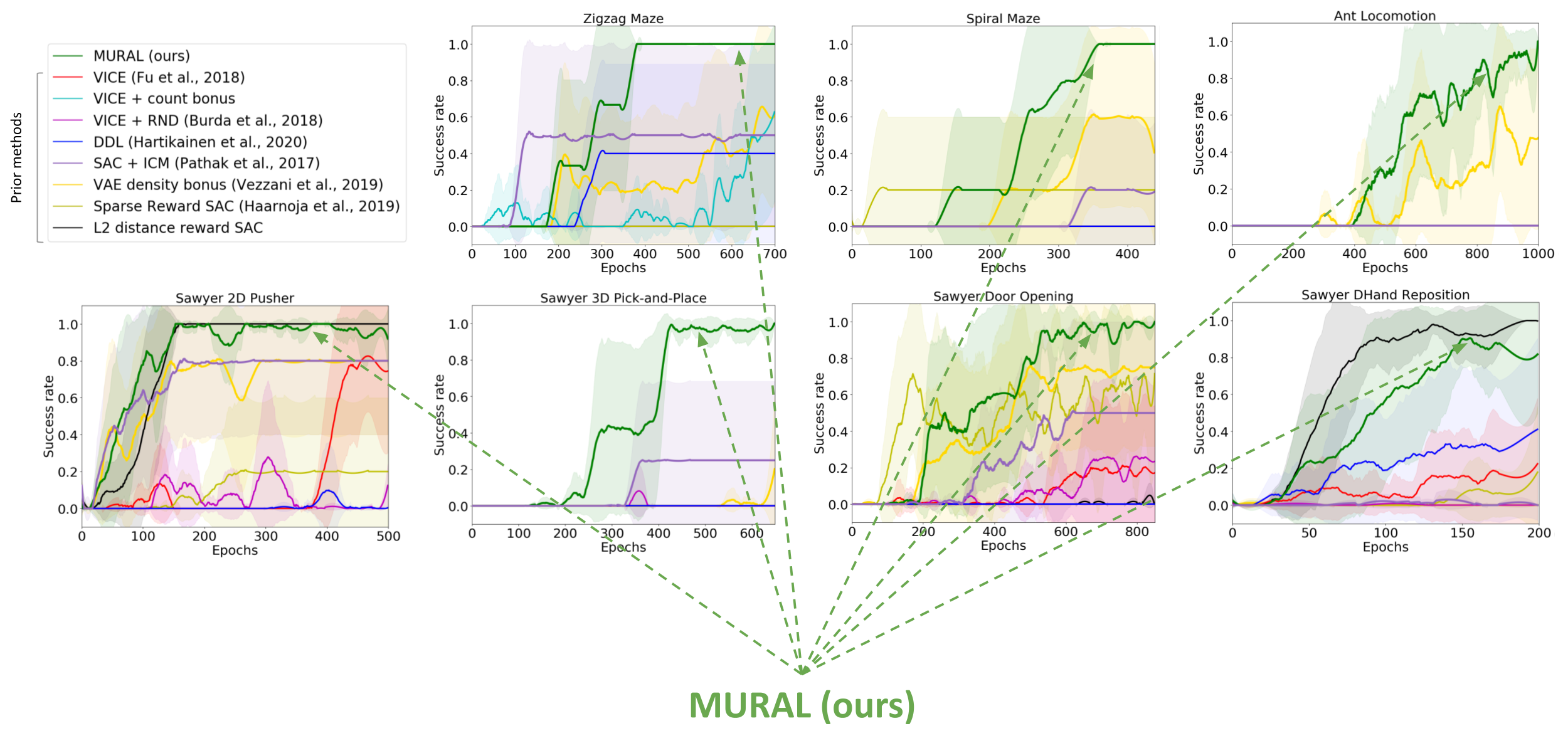Quantitative comparison of MURAL to baselines. MURAL is able to outperform baselines which perform task-agnostic exploration, standard maximum likelihood classifiers.

This suggests that using meta-NML based classifiers for outcome driven RL provides us an effective way to provide rewards for RL problems, providing benefits both in terms of exploration and directed reward shaping.

# Takeaways

In conclusion, we showed how outcome driven RL can define a class of more tractable RL problems. Standard methods using classifiers can often fall short in these settings as they are unable to provide any benefits of exploration or guidance towards the goal. Leveraging a scheme for training uncertainty aware classifiers via conditional normalized maximum likelihood allows us to more effectively solve this problem, providing benefits in terms of exploration and reward shaping towards successful outcomes. The general principles defined in this work suggest that considering tractable approximations to the general RL problem may allow us to simplify the challenge of reward specification and exploration in RL while still encompassing a rich class of control problems.

This post is based on the paper “MURAL: Meta-Learning Uncertainty-Aware Rewards for Outcome-Driven Reinforcement Learning”, which was presented at ICML 2021. You can see results on our website, and we provide code to reproduce our experiments.